Admission Now | Subjects | Classes | Eligibility | Fee-Structure | Site-Map | Available Seats | Exams | Time-Table | Rules | NOTICE BOARD | Attendance | Contact Us
NCERT Class 9 Physics Notes : WORK AND ENERGY : Mandakini Study Institute - Patna
1 / 32 / 33 / 3## WORK AND ENERGY

1.Work Done By A Constant Force

• Work is a scalar quantity equal to the product of the displacement x and the component of the force Fx in the direction of the displacement..

• Work is defined as a force acting upon an object to cause a displacement

• Mathematically, work can be expressed by the following equation.

• W= F x d cos q ( cos 0o = 1)

• where F = force, d = displacement, and the angle (theta) is defined as the angle between the force and the displacement vector

• Three things are necessary for the performance of work

• There must be an applied force F.

• There must be a displacement x.

• The force must have a component along the displacement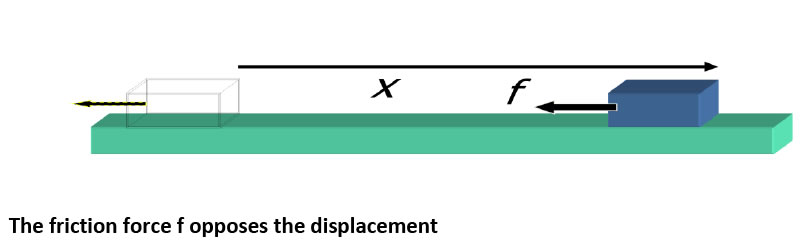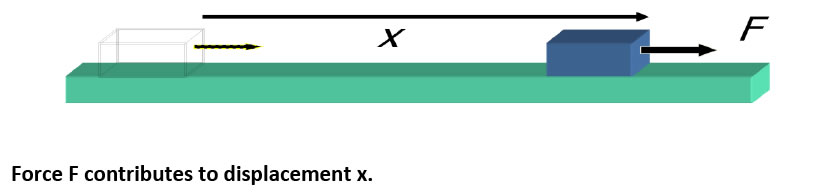### Test Yourself:

1.Calculate Work when F= 40 N and x = 4 m.

2.Calculate Work when F = -10 N and x = 4 m.

3. A lawn mower is pushed a horizontal distance of 20 m by a force of 200 N directed at an angle of 30o with the ground. What is the work of this force?

4. A student lifts a 50 pound (lb) ball 4 feet (ft) in 5 seconds (s). How many joules of work has the student completed?

### 2.Energy And Its Forms

#### James Joule

The metric system unit of energy is the joule (J), after James Joule.

• Mechanical energy is the energy which is possessed by an object due to its motion or its stored energy of position

### Forms of Energy

• Kinetic energy : is the energy of motion
Energy which a body possesses because of its motion, which occurs anywhere from an atomic level to that of a whole organism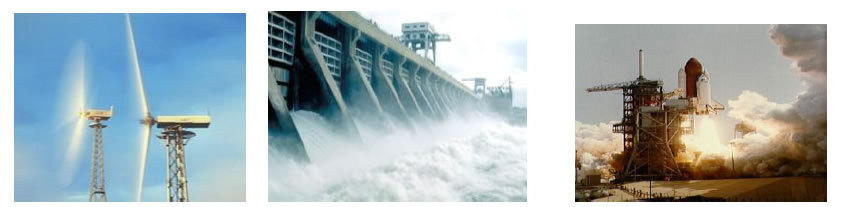Examples of Kinetic Energy: This is not an all-inclusive list.

• Electrical – The movement of atoms

• Electromagnetic or Radiant – The movement of waves

• Thermal or Heat – The movement of molecules

• Motion – The movement of objects

• Sound – The movement through waves
Engineers generally refer to thermal/heat energy as “internal energy” and use “kinetic energy” strictly in reference to motion.
Potential Energy (Stored energy or gravitational energy)

• The capacity to do work by virtue of position or configuration

• an object can store energy as the result of its position or elastic source

• Potential Energy is maximum at the maximum HEIGHT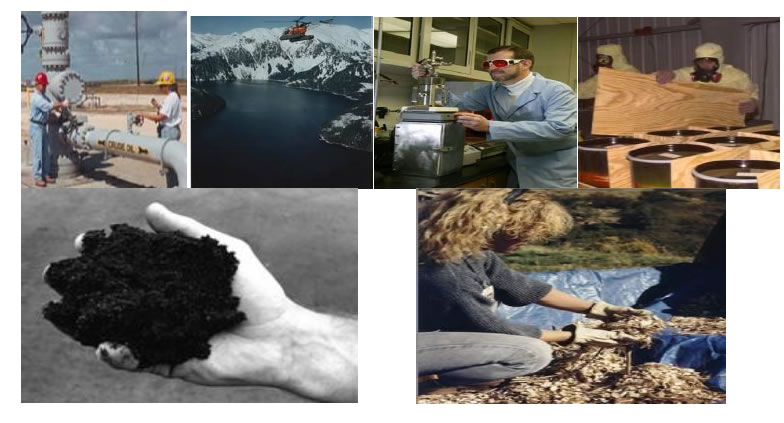Energy transformation involves the conversion of one form of energy into another form.
Examples of energy transformation include:

• Chemical – Food is consumed and converted into motion for playing sports or taking a test.

• Radiant – Sunlight is consumed by plants and converted into energy for growth

• Electrical – Energy transferred to an oven is converted to thermal energy for heating our food.

Now you know the basic forms of energy. The next question is “What are the energy sources?”
There are renewable and nonrenewable sources of energy. A renewable energy source is a form of energy that is constantly and rapidly replenished by natural processes.
Examples of renewable energy sources include:

• Biomass – The use of a living or once living organism as fuel

• Hydropower – The energy produced from the movement of water

• Geothermal – The use of heat from within the Earth or from the atmosphere near oceans to warm houses or other buildings

• Wind – The use of wind to generate electricity

Solar – The use of the sun as a source of heat; for instance, to heat a room within a house, etc.

Energy Conversion

Examples

Fossil fuels Chemical → Heat → Mechanical → Electrical

Solar cells Sunlight → Electrical

Wind turbines Kinetic → Mechanical → Electrical

Hydroelectric Gravitational potential → Mechanical → Electrical

Nuclear       Nuclear → Heat → Mechanical → Electrical

### Test Yourself

1. How much potential energy is lost by a 5Kg object to kinetic energy due a decrease in height of 4.5 m.

3. Potential energy of an object at a height
An object increases its energy when raised through a height.
The potential energy of an object at a height depends on the ground level or the zero level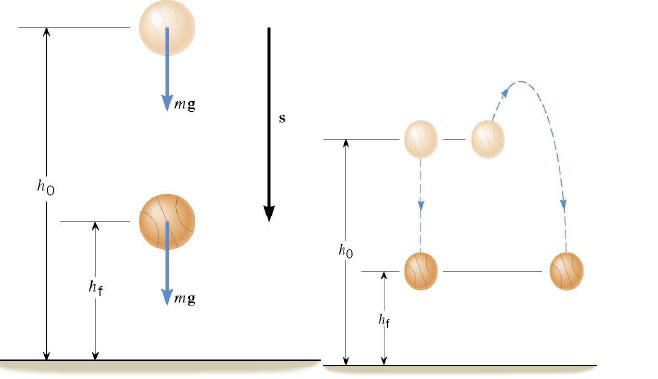#### 4. Law Of Conservation Of Energy

The principle of Conservation of Mechanical Energy
The total mechanical energy (E=KE+PE) of an object remains constant as the object moves, provided that the net work done by external non-conservative forces is zero, Wnc=0J
Total mechanical energy: the sum of kinetic energy and gravitational potential energy

E = KE + PE
Wnc = (KEf - KEo) + (PEf - PEo)
Wnc = (KE+ PEf) - (KEo + PEo)
Wnc = Ef - Eo
Ef = KEf + PEf) Eo = KEo + PEo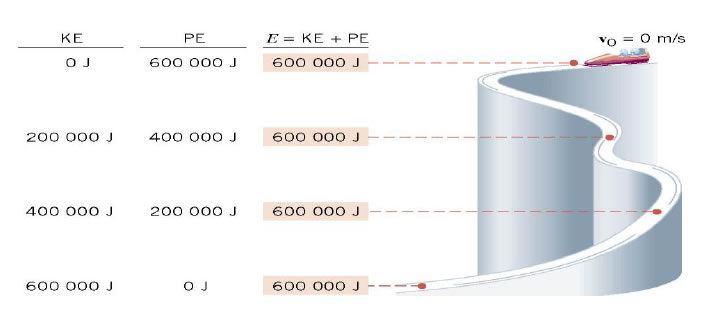5. Rate of Doing Work & Commercial Unit Of Energy POWER
Rate at which work is performed or energy is expended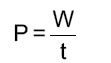Watt is the base unit of Power

One watt is equal to 1 joule of work per second
Types of Power

• Electrical Power
Uses electrical energy to do work

• Mechanical Power
Uses mechanical energy to do work (linear, rotary)

• Fluid Power
Uses energy transferred by liquids (hydraulic) and gases (pneumatic)

• Power is the rate that we use energy.

• Power = Work or Energy / Time

• P = W/t = F x d/t = F v

• The unit joule is too small .The bigger unit of energy called kilowatt hour (kW h)
1 kW h is the energy used in one hour
at the rate of 1000 J s–1 (or 1 kW).

1 kW h = 1 kW *1 h

= 1000 W*3600 s

= 3600000 J

1 kW h = 3.6 x 106 J. WP=t

### Test Yourself

1. A 5 Kg Cart is pushed by a 30 N force against friction for a distance of 10m in 5 seconds. Determine the Power needed to move the cart.

2. A student lifts a 50.0 pound (lb) ball 4.00 feet (ft) in 5 .00seconds (s). How many watts of power are used to lift the ball?
Important Points for Work Problems:

• Always draw a free-body diagram, choosing the positive x-axis in the same direction as the displacement.

• Work is negative if a component of the force is opposite displacement direction

• Work done by any force that is at right angles with displacement will be zero (0).

• For resultant work, you can add the works of each force, or multiply the resultant force times the net displacement.

• Energy is the ability to move

• Potential is stored energy (Statics)

• Dependant on height

• Kinetic is moving energy (Dynamics)

• Dependant on velocity

• Springs store energy dependant on distance and constant

### QUESTION BANK

#### One mark questions

1. Does work have a direction?

2. Does the kinetic energy of an object depend on its direction of motion?

3. Cam matter be converted into energy?

4. Give an example of conversion of chemical energy into heat energy.

#### Two marks questions

1. Two persons do the same amount of work. The first person does it in 10 s and the second, in 20 s.Find the ratio of the power used by the person to that by the second person.

2. A body of mass 25 g has a momentum of 0.40 kgm/s.Find its kinetic energy.

3. Define work and write its units.

4. By what factor does the kinetic energy of an object depend on its direction of motion?

#### Three marks questions

1. How much time will it take to perform 440 j of work at a rate of 11 W.

2. A body of mass 3.0kg and a body B of mass 10 kg are dropped simultaneously from a height of 14.9m.Calculate their Momenta, their Potential energies and kinetic energies when they are 10m above the ground.

3. lA man does 200j ofl work in 10 seconds and a boy does 100j of work in 4 seconds. Who is delivering more power? Find the Ratio of power delivered by the man to that by the boy.

#### Five marks questions

1. Show that the work done by a force is given by the product of the force and the projection of the displacement along the force.

2. Find the expression for gravitational potential energy of a body of mass m at height h.

3. Why does a person standing for a long time get tired when he does not appear to do any work?

4. How can you justify that a body kept at a greater height has larger energy?